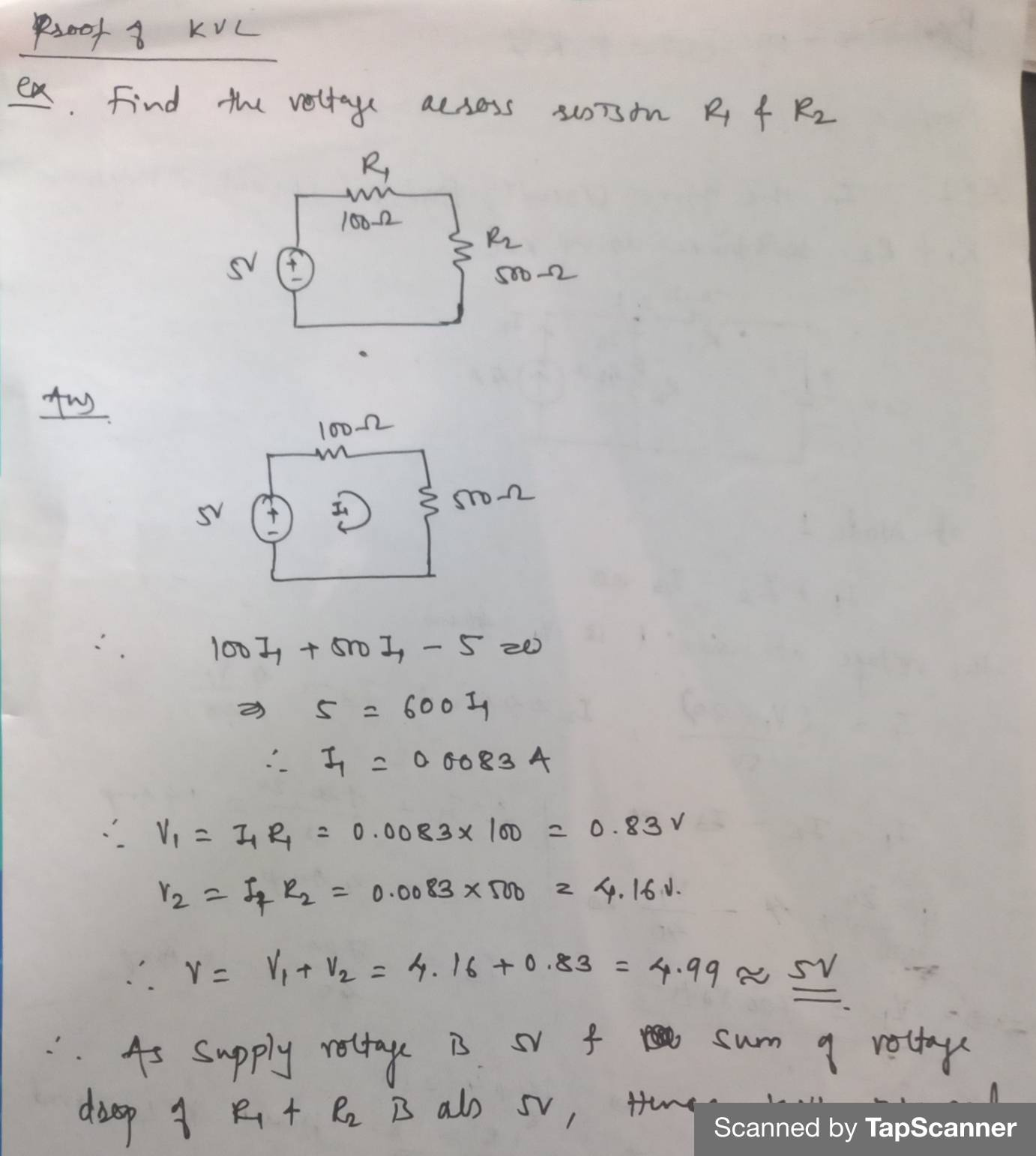### Basic Laws

Kirchhof’s Voltage Law:
It states that, “The algebraic sum of all the voltages entering and leaving at the node must be equal to zero or the algebraic sum of all the voltages at loop must be zero”.
This law deals with conservation of energy around a closed path circuit.
Derivation of KVL
Using Maxwell’s equation,
▽.E = ९/ε0   ▽.B=0   ▽xE= - ∂B/∂t   ▽xB= μJ
Now,

▽xE= - ∂B/∂t
∮▽xE = - d/dt∮B.ds
∮ E.ds = -d/dt ØB
as ØB= BA cosӨ and no change in B, A and Ө  ØB=0
Therefore,  ΣV=0
Considering V1 and V2 are entering and V3 and V4 leaving the circuit, then
V1+V2=V3+V4